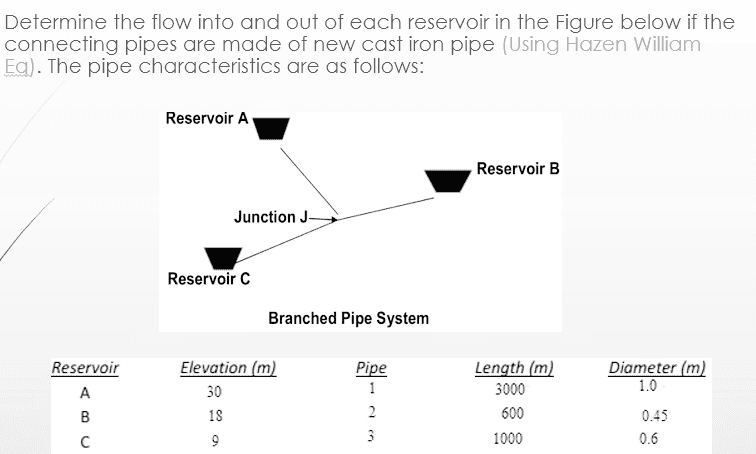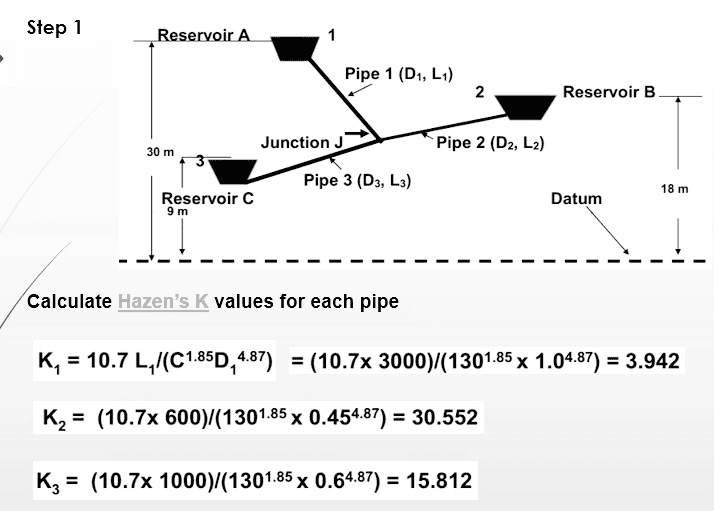# Flow in pipe

foo9008

## Homework Statement

in this network of pipe , the author make the attempts to solve it using ( Q1 = Q2 + Q3) , so delta Q = Q1 -(Q2 +Q3) , the value of head of junction that he gt is 25.2 , with delta Q = (3x10^-3) ( not shown in the working)

## The Attempt at a Solution

however, when i tried to solve it using ( Q1 + Q2 = Q3) , which means ( Q1 +Q2 - Q3 ) , thae ans that i gt is hj = 28 , with delta Q = 0.07 [/B]

P/s : we have to find the delta Q = 0 for the ans so that water from inlet = water from outlet
so , question has two ans depending on the flow of water ??? or i am wrong ?

## Answers and Replies

foo9008foo9008
is it wrong to have 2 answer for such question ,
i find it work by using ( Q1 = Q2 + Q3) and ( Q1 + Q2 = Q3)

foo9008
Anyone can comment ?

foo9008
bump

foo9008
deleted

foo9008
Bump

foo9008
i have done this question in 2 ways , which is ( Q1 - (Q2 + Q3) ) and ( Q1 +Q2 - Q3 ) which is correct ? or both are correct ? please refer to post 1

foo9008
pls ignore the question i posted above ....
now , i have new question with the same figure..
from the diagram , we know that the junction is lower than the pipe at B . why the author wanna make an assumption that the junction is located at 20m from the datum ? is it correct to do so ?

foo9008
can i start a new thread and delete this thread ? how to delete this thread ??